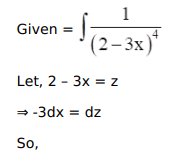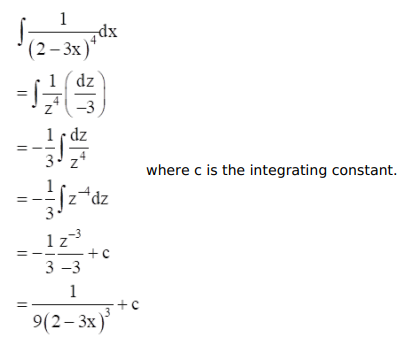# Mark against the correct answer in each of the following:

Question:

Mark $(\sqrt{)}$ against the correct answer in each of the following:

$\int \frac{1}{(2-3 x)^{4}} d x=?$

A. $\frac{1}{15(2-3 x)^{5}}+C$

B. $\frac{1}{-12(2-3 x)^{3}}+C$

C. $\frac{1}{9(2-3 x)^{3}}+C$

D. none of these

Solution: